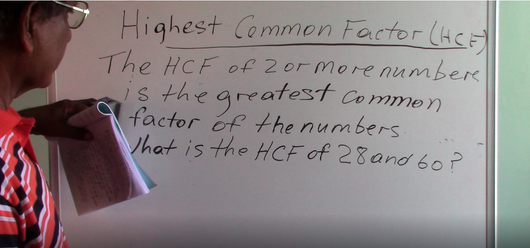# Grade 6 & 7 - Highest Common Factor (Introduction)

Regular price \$2.00 Sale

A 4 and 1/2 math tutorial on Highest Common Factor (Introduction) for Grade 6 & 7. Tutorial done by M. Chan (Trained Certified Teacher).

Highest Common Factor of HCF. The HCF of 2 or more Numbers is the greatest common factor of the numbers. A Number will have more than 1 factor, so we are looking at the greatest common factor. If there are 2 or 3 numbers, the HCF must be in all of these numbers and common in all of them. In this example, we are going to find the HCF of 28 and 60...